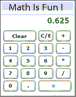How do you change a fraction to a decimal?

196 views
asked Mar 19, 2013 in Math

You need to divide the numerator (top part) by the denominator (bottom part). I would just punch the numbers in a calculator for an answer, but you can do it by hand using long division and carrying out the decimal points.
answered Mar 19, 2013 by (61,690 points)
edited Mar 20, 2013 by whizkid
thank you very much!
+1 vote

You divide the numerator by the denomenator to get a decimal.Sometimes the number keeps on going for a long time.

You could also find how many times the denomenator goes into 100. You multiply that number by the numerator.

answered Mar 24, 2013 by (30,500 points)
edited Mar 26, 2013 by smile828
+1 vote

You divide the numerator by the denominator. You can use a calculator, or do it by hand, just carry out the decimal points.

answered Mar 25, 2013 by (16,950 points)
+1 vote

It's long sorry:

The simplest method is to use a calculator.

Just divide the top of the fraction by the bottom, and read off the answer !Example: What is 5/8 as a decimal ... ?

... get your calculator and type in "5 / 8 ="

The answer should be 0.625

To convert a Fraction to a Decimal manually, follow these steps:

 Step 1: Find a number you can multiply by the bottom of the fraction to make it 10, or 100, or 1000, or any 1 followed by 0s. Step 2: Multiply both top and bottom by that number. Step 3. Then write down just the top number, putting the decimal point in the correct spot (one space from the right hand side for every zero in the bottom number)

Source: mathsisfun.com.

answered Apr 16, 2013 by (2,130 points)
edited Apr 17, 2013 by Netball